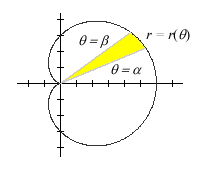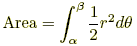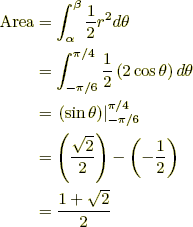index: click on a letter A B C D E F G H I J K L M N O P Q R S T U V W X Y Z A to Z index index: subject areas numbers & symbols sets, logic, proofs geometry algebra trigonometry advanced algebra & pre-calculus calculus advanced topics probability & statistics real world applications multimedia entrieswww.mathwords.com about mathwords website feedback

Area Using Polar Coordinates
Polar Integral Formula

The area between the graph of r = r(θ) and the origin and also between the rays θ = α and θ = β is given by the formula below (assuming α β).

 Formula:Example: Find the area of the region bounded by the graph of the lemniscate r2 = 2 cos θ, the origin, and between the rays θ = –π/6 and θ = π/4.